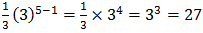Definition Of Geometric Sequence

Geometric sequence is a sequence in which each term after the first term a is obtained by multiplying the previous term by a constant r, called the common ratio. It is obvious that a ≠ 0 and r ≠ 0 or 1

Example: 1, 2, 4, 8, 16, 32, . . . is a geometric sequence
Each term of this geometric sequence is multiplied by the common ratio 2

The general form of a geometric sequence with first term a and common ratio r is
a, ar, ar2, ar3 ................. ar(n-1)
The general term or nth term of a geometric sequence is ar(n-1)
Geometric series is the indicated sum of the terms of a geometric sequence.
For the geometric sequence 1, 2, 4, 8, 16, 32, the corresponding geometric series is1 + 2 + 4 + 8 + 16 + 32

Solved Examples on Geometric Sequence

Ques: Which of the following is a geometric sequence?

Choices:

A. 2, 4, 8, 16, 30, 32,......
B. 2, 4, 6, 8, 16, 32, 64,....
C. 2, 4, 8, 12, 18, 24,......
D. 2, 4, 8, 18, 36, 64,.....

Solution:

Step1: Geometric sequence is a sequence in which each term after the first term is obtained by multiplying the previous term by a constant
Step 2: 2, 4, 6, 8, 16, 32, 64,...... is the only sequence in the options in which each term is obtained by multiplying the previous term by 2

Ques: Find the 5th term of the geometric sequence 1/3,1,3.......

Choices:

A. 9
B. 3
C. 81
D. 27

Step 3: The 5th term is[Substitute and simplify.]